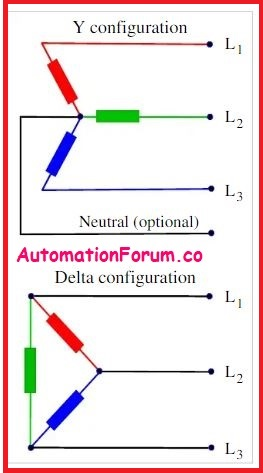# Difference between Two Phase and Three Phase## What is Two Phase?

Two-phase electrical power was an optional process (polyphase) alternating current electric power distribution technology used in the early twentieth century. Two circuits were employed, with voltage phases that differed by a quarter of a cycle, or 90 degrees. Typically, four wires were utilised in circuits, two for each phase. Three wires were used less commonly, using a common wire with a larger-diameter conductor. Two entire rotor and field assemblies were used in two-phase generators, with windings physically separated to create two-phase current.

There are two stages to 2 phase electric power (that supply electricity in a building):

• In this system, there are usually four wires. There are two wires in each phase.
• In some two-phase systems, three wires are present. Each phase has its separate connected wire, while the common wire has its separate wire.
• The phases (electricity carriers) are organised in a 90-degree symmetrical pattern.
• Three wires be used to build two-phase systems (two “hot” plus a common neutral).

## The function of the 2 phase current:

There were two circuits used, each having voltage phases that diverged by a half of a cycle, or 90 degrees. From the phase voltage VP can be determined for each phase. Similarly, the line voltage VL can also be determined from square root of 2- phase voltage.

## What are the features of 2 phase current?

• When the single-phase power system became ineffective, the two-phase power system was developed.
• The self-starting motors are primarily powered by the two-phase electric system.
• Two phase current feature is that, unlike three-phase current, they may give electricity at a consistent rate without variations.
• Two-phase circuits have the added benefit of delivering continuous combined power to an ideal load.

## Where 2 phase current can be applied?

• Structures that demand less than 10 kilowatts of energy are best served by two-phase power systems.
• The winding architecture of induction motors built for two-phase operation is identical to that of capacitor start single-phase motors.

## What is Three Phase?

A typical technique of alternating-current electric power generation, transmission, and distribution is three-phase electric power. It is a form of polyphase system that is utilised by electrical grids all over the world to transfer power. Large motors and other heavy loads are also powered by it. Because it utilises less conductor material to transfer electrical power, a three-phase system is usually more cost effective than an identical single-phase or two-phase system at the same line to ground voltage.

## What is the function of the three phase current?

Three conductors carry an alternating current of the same frequency and voltage amplitude relative to a common reference in a symmetric three-phase power supply system, but with a phase difference of one third the period. The common reference is frequently connected to ground and, in some cases, to the neutral, a current-carrying wire. The voltage on any conductor reaches its peak one third of a cycle after one of the other conductors and one third of a cycle before the remaining conductor due to the phase difference. A balanced linear load receives constant power transmission due to this phase delay. It also enables the creation of a spinning magnetic field in an electric motor and the generation of other phase arrangements through the use of transformers.

The amplitudes of line currents are 3 times those of phase currents for ∆ -connected three-phase source, and the line voltage is the same as the phase voltage.

The amplitudes of line voltages are 3 times that of phase voltages, and the line current is the same as the phase current in a Y-connected three-phase source.

## What are the features of 3 phase current?

• The load of all electric types of equipment is evenly distributed among the three phases.
• Vibrations in the power are also reduced since the current’s load is balanced.

## Where 3 phase current can be applied?

• A three-phase power supply is used
• To manage companies
• To power three-phase equipment
• Trains will be supplied with overhead power – those overhead cables you see above the tracks are all connected to any of the three phases and separated from others by neutral connection
• To provide households with electricity